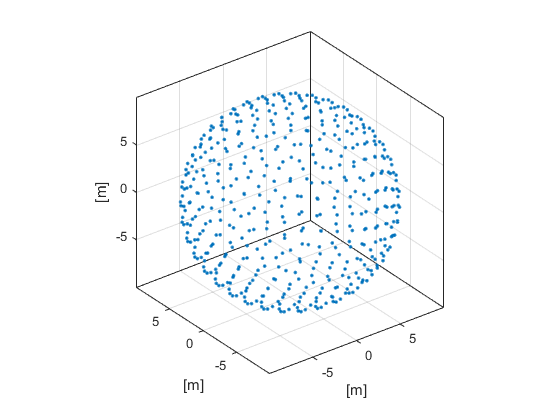# makeCartSphere

Create a 3D Cartesian sphere.

## Syntax

```sphere = makeCartSphere(radius, num_points)
sphere = makeCartSphere(radius, num_points, center_pos)
sphere = makeCartSphere(radius, num_points, center_pos, plot_sphere)
```

## Description

`makeCartSphere` creates a 3 x `num_points` array of the Cartesian coordinates of points evenly distributed over a sphere using the Golden Section Spiral method. The function is based on code by Patric Boucher and Henry Bland on http://www.xsi-blog.com. The 3D plot that is displayed after calling `makeCartSphere(10, 500, [0,0,0], true);` is given below.## Inputs

 `radius` sphere radius [m] `num_points` number of points on the sphere

## Optional Inputs

 `center_pos` [x, y, z] position of the circle center [m] (default = [0, 0, 0]) `plot_sphere` Boolean controlling whether the Cartesian coordinates are plotted using `plot3` (default = false)

## Outputs

 `sphere` 3 x `num_points` array matrix of Cartesian points

## Examples

`cart2grid`, `makeCartCircle`, `makeSphere`